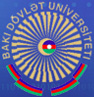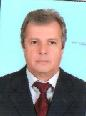## Orujov Hamzaga Davud oghlu

D.Sci. in Mathematics & Physics, professorWork phone number: +(994) 12 438 25 18
e-mail: hamzaga@yahoo.com

PERSONAL DATA
He was born February 1, 1949 in Shemakha.
In 1966 graduated from the secondary school
In 1967 has entered the mechanical-mathematical faculty of Baku State University, which has graduated in a 1972, in specialty of "Mathematics"
1972-1975-postgraduate student of Institute of Mathematics and Mechanics of ANAS
1975-1978 -junior scientific worker in Institute of Mathematics and Mechanics of ANAS
1979-1980- senior scientific worker in Institute of Mathematics and Mechanics of ANAS
From 1986 to 1995 worked as associate professor at BSU
Since 1995 he is professor at BSU
Married, has 4 children

1967-1972, student, Mechanical-mathematical faculty of Baku State University
1976, candidate of physical-mathematics sciences, Thesis: «Investigation of self-adjoint extension of higher order operator differential expressions»
1995, doctor of candidate of physical-mathematics sciences, Thesis: «Boundary-value problems for operator-differential equations»

PROFESSIONAL BACKGROUND
1975-1978 -junior scientific worker in Institute of Mathematics and Mechanics of ANAS
1979-1980- senior scientific worker in Institute of Mathematics and Mechanics of ANAS
From 1986 to 1995 worked as docent at BSU
Since 1995 he is professor at BSU
He has delivered such courses of lectures as "Geometry and Algebra", "Applied Functional Analysis", "Approximating solution of differential equations"
He is the author of 43 scientific works, textbooks

RESEARCH INTERESTS
Solution of differential operator equations and spectral problems for such equations

INTERNATIONAL CONFERENCES, SYMPOSIUMS
Russia (Novgorod-1976, Novosibirsk-1980), Ukraine (Chernovtsi-1989, Lvov-1992, Kiev-1992), Moscow-1993, Hamburg-1995, Shiraz-1996, Tabriz-1997, Kiev-2001

LIST OF SELECTED PUBLICATIONS
1. Smoothness of generalized solutions of differential equations in Hilbert space //. Transactions of NAS of Ukrain, num.7, 1989, vol. А., pp. 17-20
2. Polynomial approximation of solution of Cauchy problem for hyperbolic differential-operator equation // Transactions of NAS of Ukraine, num.10, 1992 г., pp. 10-12
3. On polynomial approximation of solutions of differential-operator equations // Ukrainian Math. Journal, vol. 45, num.3, 1993., pp. 140-142
4. On rate of convergence of polynomial approximation of solution of Cauchy problem for differential-operator equations // Transactions of NAS of Ukraine, num.9, 1993 , pp. 14-17
5. Polynomial approximation of solutions of some differential equations // Russian Mathematical Surveys, vol. 48, issue. 4/292/, 1993, p. 190.
6. On solvability of boundary value problems for abstract polyharmonic equations // Transactions of NAS of Russia, vol. 334, num.3, 1994 г., pp. 281-283.
7. Polynomial approximations of solutions of higher-order operator-differential equations // Ukrainian Math. Journal, vol. 46, num.5, 1994, pp. 952-955.
8. Approximate solution of the Dirichlet problem for the polyharmonic equation on the semi axis // International conference "Application of Information-Communication Technologies in Science and Education", Baku, 2004, september, p.152
9. On polynomial approximation of solution of Cauchy problem for operator-differential equation // "International Workshop on Analysis and its applications" Mersin-Turkey, september, 2004, p. 43.
10. Polynomial approximation of solution of Cauchy problem for operator- differential equation // International Scientific conference "Mathematical analysis, differential equations and their applications", september 18-23, 2006, Uzhgorod, Ukraine, pp. 166-167
11. The Cauchy problem for the abstract Euler-Poisson-Darboux equation // 2nd International Conference "Application of Information-Communication Technologies in Science and Education" , Articles (Book 2) Baku, 01-03 november, 2007, p. 403-407
12. The spectral problem for abstract biharmonic equation // Science Conference, devoted to the 85th anniversary from the date of a birth of national leader Haydar Aliyev "Modern problems of mathematics, informatics and economics", Baku, 26th april, 2008, p. 108
13. Application of the finite inteqral transformation method to solving mixed problems for hyperbiolic equations with irregular boundary conditions. //Proceedings of the Institute of Mathematics and Mechanics, National Academy of Sciences of Azerbaijan, 2014, vol. 40, Special Issue, p.p. 375-385

BOOKS
Linear Algebra (Problems), Textbook, Baku, 2002